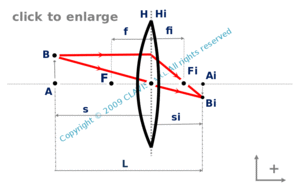and orgeometrical optics
paraxial conjugation

# thin lens for a given magnification and a given distance between conjugated planes

Caution
Fill in all input cells.
Choose all parameters unit.
Magnification and distances are algebric.
Positive directions are according the arrows in the bottom right of the scheme.

InputValueunit
a
m
OutputValueUnit
fto be calculated
fito be calculated
sto be calculated
sito be calculateda : distance from the object plane to the image plane s : distance from the lens to the object plane si : distance from the lens to the image plane

Note
This calculation page can not detect all unrealistic inputs.